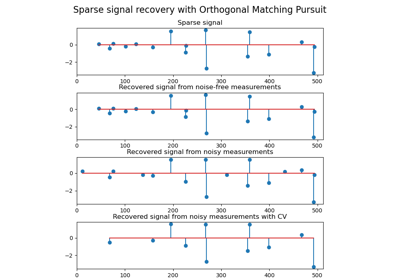# sklearn.linear_model.OrthogonalMatchingPursuitCV¶

class sklearn.linear_model.OrthogonalMatchingPursuitCV(*, copy=True, fit_intercept=True, normalize='deprecated', max_iter=None, cv=None, n_jobs=None, verbose=False)[source]

Cross-validated Orthogonal Matching Pursuit model (OMP).

See glossary entry for cross-validation estimator.

Read more in the User Guide.

Parameters:
copybool, default=True

Whether the design matrix X must be copied by the algorithm. A false value is only helpful if X is already Fortran-ordered, otherwise a copy is made anyway.

fit_interceptbool, default=True

Whether to calculate the intercept for this model. If set to false, no intercept will be used in calculations (i.e. data is expected to be centered).

normalizebool, default=False

This parameter is ignored when fit_intercept is set to False. If True, the regressors X will be normalized before regression by subtracting the mean and dividing by the l2-norm. If you wish to standardize, please use StandardScaler before calling fit on an estimator with normalize=False.

Changed in version 1.2: default changed from True to False in 1.2.

Deprecated since version 1.2: normalize was deprecated in version 1.2 and will be removed in 1.4.

max_iterint, default=None

Maximum numbers of iterations to perform, therefore maximum features to include. 10% of n_features but at least 5 if available.

cvint, cross-validation generator or iterable, default=None

Determines the cross-validation splitting strategy. Possible inputs for cv are:

• None, to use the default 5-fold cross-validation,

• integer, to specify the number of folds.

• An iterable yielding (train, test) splits as arrays of indices.

For integer/None inputs, KFold is used.

Refer User Guide for the various cross-validation strategies that can be used here.

Changed in version 0.22: cv default value if None changed from 3-fold to 5-fold.

n_jobsint, default=None

Number of CPUs to use during the cross validation. None means 1 unless in a joblib.parallel_backend context. -1 means using all processors. See Glossary for more details.

verbosebool or int, default=False

Sets the verbosity amount.

Attributes:
intercept_float or ndarray of shape (n_targets,)

Independent term in decision function.

coef_ndarray of shape (n_features,) or (n_targets, n_features)

Parameter vector (w in the problem formulation).

n_nonzero_coefs_int

Estimated number of non-zero coefficients giving the best mean squared error over the cross-validation folds.

n_iter_int or array-like

Number of active features across every target for the model refit with the best hyperparameters got by cross-validating across all folds.

n_features_in_int

Number of features seen during fit.

New in version 0.24.

feature_names_in_ndarray of shape (n_features_in_,)

Names of features seen during fit. Defined only when X has feature names that are all strings.

New in version 1.0.

orthogonal_mp

Solves n_targets Orthogonal Matching Pursuit problems.

orthogonal_mp_gram

Solves n_targets Orthogonal Matching Pursuit problems using only the Gram matrix X.T * X and the product X.T * y.

lars_path

Compute Least Angle Regression or Lasso path using LARS algorithm.

Lars

Least Angle Regression model a.k.a. LAR.

LassoLars

Lasso model fit with Least Angle Regression a.k.a. Lars.

OrthogonalMatchingPursuit

Orthogonal Matching Pursuit model (OMP).

LarsCV

Cross-validated Least Angle Regression model.

LassoLarsCV

Cross-validated Lasso model fit with Least Angle Regression.

sklearn.decomposition.sparse_encode

Generic sparse coding. Each column of the result is the solution to a Lasso problem.

Notes

In fit, once the optimal number of non-zero coefficients is found through cross-validation, the model is fit again using the entire training set.

Examples

>>> from sklearn.linear_model import OrthogonalMatchingPursuitCV
>>> from sklearn.datasets import make_regression
>>> X, y = make_regression(n_features=100, n_informative=10,
...                        noise=4, random_state=0)
>>> reg = OrthogonalMatchingPursuitCV(cv=5).fit(X, y)
>>> reg.score(X, y)
0.9991...
>>> reg.n_nonzero_coefs_
10
>>> reg.predict(X[:1,])
array([-78.3854...])


Methods

 fit(X, y) Fit the model using X, y as training data. get_params([deep]) Get parameters for this estimator. Predict using the linear model. score(X, y[, sample_weight]) Return the coefficient of determination of the prediction. set_params(**params) Set the parameters of this estimator.
fit(X, y)[source]

Fit the model using X, y as training data.

Parameters:
Xarray-like of shape (n_samples, n_features)

Training data.

yarray-like of shape (n_samples,)

Target values. Will be cast to X’s dtype if necessary.

Returns:
selfobject

Returns an instance of self.

get_params(deep=True)[source]

Get parameters for this estimator.

Parameters:
deepbool, default=True

If True, will return the parameters for this estimator and contained subobjects that are estimators.

Returns:
paramsdict

Parameter names mapped to their values.

predict(X)[source]

Predict using the linear model.

Parameters:
Xarray-like or sparse matrix, shape (n_samples, n_features)

Samples.

Returns:
Carray, shape (n_samples,)

Returns predicted values.

score(X, y, sample_weight=None)[source]

Return the coefficient of determination of the prediction.

The coefficient of determination $$R^2$$ is defined as $$(1 - \frac{u}{v})$$, where $$u$$ is the residual sum of squares ((y_true - y_pred)** 2).sum() and $$v$$ is the total sum of squares ((y_true - y_true.mean()) ** 2).sum(). The best possible score is 1.0 and it can be negative (because the model can be arbitrarily worse). A constant model that always predicts the expected value of y, disregarding the input features, would get a $$R^2$$ score of 0.0.

Parameters:
Xarray-like of shape (n_samples, n_features)

Test samples. For some estimators this may be a precomputed kernel matrix or a list of generic objects instead with shape (n_samples, n_samples_fitted), where n_samples_fitted is the number of samples used in the fitting for the estimator.

yarray-like of shape (n_samples,) or (n_samples, n_outputs)

True values for X.

sample_weightarray-like of shape (n_samples,), default=None

Sample weights.

Returns:
scorefloat

$$R^2$$ of self.predict(X) wrt. y.

Notes

The $$R^2$$ score used when calling score on a regressor uses multioutput='uniform_average' from version 0.23 to keep consistent with default value of r2_score. This influences the score method of all the multioutput regressors (except for MultiOutputRegressor).

set_params(**params)[source]

Set the parameters of this estimator.

The method works on simple estimators as well as on nested objects (such as Pipeline). The latter have parameters of the form <component>__<parameter> so that it’s possible to update each component of a nested object.

Parameters:
**paramsdict

Estimator parameters.

Returns:
selfestimator instance

Estimator instance.

## Examples using sklearn.linear_model.OrthogonalMatchingPursuitCV¶Orthogonal Matching Pursuit

Orthogonal Matching Pursuit